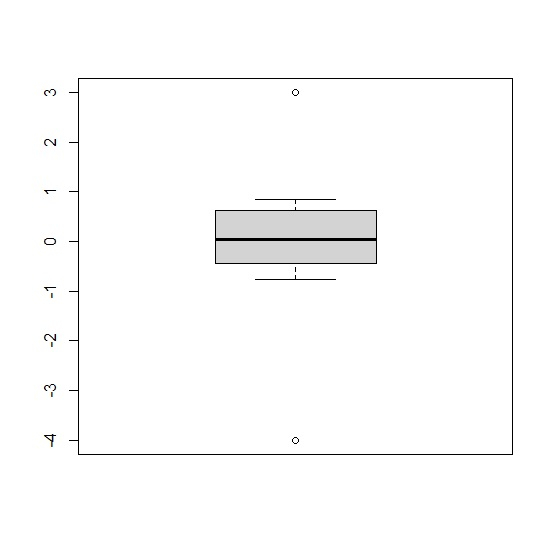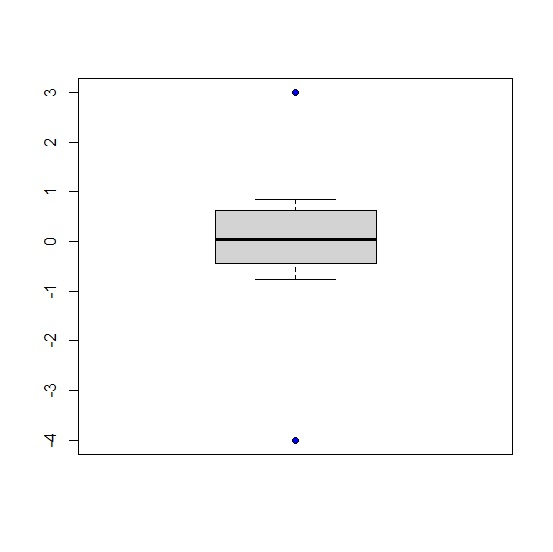# How to fill the outliers with different color in base R boxplot?

To fill the outliers in boxplot with different color in base R, we can use outpch argument for the shape and outbg argument for colors.

For example, if we have a vector called X that contains some outliers then we can create a boxplot of X with different color outliers by using the below mentioned command −

boxplot(X,outpch=21,outbg="blue")

## Example

To fill the outliers in boxplot with different color in base R, use the code given below −

x<-c(-4,rnorm(5),3)
boxplot(x)

## Output

If you execute the above given code, it generates the following output −To fill the outliers in boxplot with different color in base R, use the code given below −

x<-c(-4,rnorm(5),3)
boxplot(x,outpch=21,outbg="blue")

## Output

If you execute the above given code, it generates the following output −